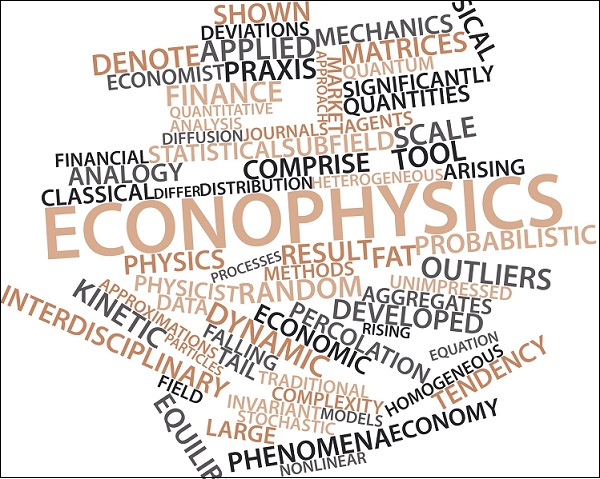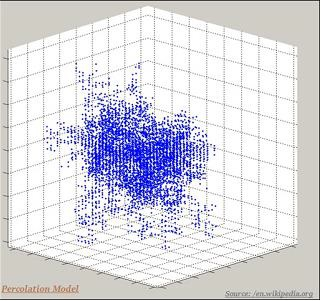# Physics - Econophysics

## Introduction

• Econophysics is an interdisciplinary science that studies the dynamic behavior of finance and economic markets.

• In order to solve the problems of economics and also to understand the dynamic behavior of the market, the econo-physicists develop applied theories.• Econophysics, sometime, is also known as the physics of finance.

• It applies statistical mechanics for the economic analysis.

## Econophysics Questions

• The econophysics questions include −

• How to accurately measure and explain the significant properties of market dynamics?

• How to stabilize the markets?

• What are the different behaviors in different markets?

## Tools of Econophysics

• The fundamental tools of econophysics are −

• Probabilistic method

• Statistical method

• These two methods are borrowed from statistical physics.

• Other tools taken from Physics

• Fluid dynamics

• Classical mechanics

• Quantum mechanics

## Models of Econophysics

• Following are the major models those are used in Econophysics −

• Percolation Model

•• Kinetic exchange models of markets

• Chaotic models

• Information theory

• Random matrix theory

• Diffusion theory